# Fraction

(diff) ← Older revision | Latest revision (diff) | Newer revision → (diff)

arithmetical

A number consisting of one or more equal parts of a unit. It is denoted by the symbol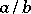, whereand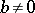are integers (cf. Integer). The numeratorofdenotes the number of parts taken of the unit; this is divided by the number of parts equal to the number appearing as the denominator. A fraction may also be considered as the ratio produced by dividingby.

The fractionremains unchanged if both the numerator and the denominator are multiplied by the same non-zero integer. Owing to this fact, any two fractionsand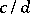may be brought to a common denominator, i.e.andmay be replaced by fractions equal toand, respectively, both of which have the same denominator. Moreover, fractions may be reduced by dividing their numerator and denominator by the same number; accordingly, any fraction may be represented as an irreducible fraction, i.e. a fraction the numerator and denominator of which have no common factors.

The sum and the difference of two fractionsand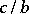having a common denominator are given by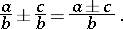In order to add or to subtract fractions with different denominators they must first be reduced to fractions with a common denominator. As a rule, the least common multiple of the numbersandis taken as the common denominator. Multiplication and division of fractions is given by the following rules: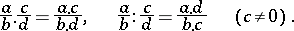A fractionis said to be a proper fraction if its numerator is smaller than its denominator; otherwise it is an improper fraction. A fraction is said to be a decimal fraction if its denominator is a power of the number 10 (cf. Decimal fraction).

## Formal definition of fractions.

Fractions may be represented as ordered pairs of integers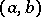,, for which an equivalence relation has been specified (an equality relation of fractions), namely, it is considered thatif. The operations of addition, subtraction, multiplication, and division are defined in this set of fractions by the following rules: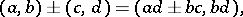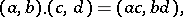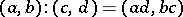(thus, division is defined only if).

A similar definition of fractions is convenient in generalizations and is accepted in modern algebra (cf. Fractions, ring of).

The set of fractions (of the integers) is denoted by. With the arithmetical operations and natural order defined in the main article above it is an ordered field. The absolute value gives a metric on. Completion ofin this metric (e.g. by using Cauchy sequences, cf. Fundamental sequence) leads to, the ordered field of real numbers (cf. Real number). In this connection, a fraction is also called a rational number, and a number fromthat is not a fraction is called an irrational number, see, e.g., [a1].
For a construction offromusing Dedekind cuts (cf. also Dedekind cut) see, e.g., [a2].
For aliquot fractions (i.e. numbers of the form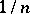,a positive integer) see Aliquot ratio.